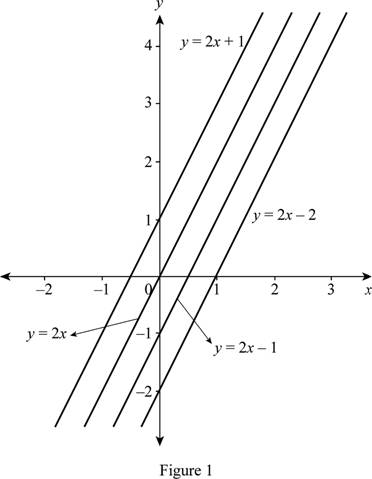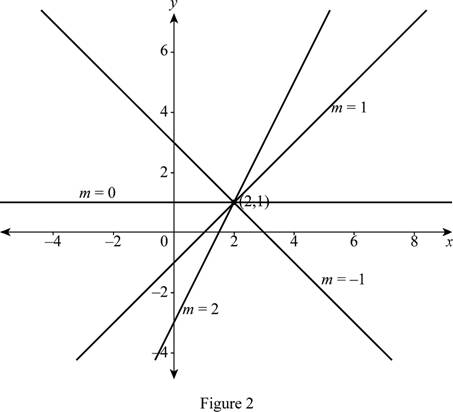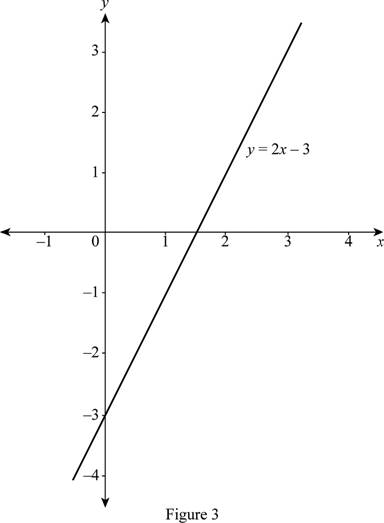# An equation of the family of linear function with slope 2 and sketch the members of the family on the graph.### Single Variable Calculus: Concepts...

4th Edition
James Stewart
Publisher: Cengage Learning
ISBN: 9781337687805### Single Variable Calculus: Concepts...

4th Edition
James Stewart
Publisher: Cengage Learning
ISBN: 9781337687805

#### Solutions

Chapter 1.2, Problem 5E

(a)

To determine

## To find: An equation of the family of linear function with slope 2 and sketch the members of the family on the graph.

Expert Solution

An equation for the family of linear function with slope 2 is f(x)=2x+c.

### Explanation of Solution

The general equation of a linear function is of the form y=mx+c with slope m and y-intercept c.

Given that, the slope (m) is 2.

Thus, the linear function of slope 2 is y=2x+c where c is any real number.

Obtain the equations for the various c values.

If c=1, then y=2x+1.

If c=0, then y=2x.

If c=1, then y=2x1.

If c=2, then y=2x2.

Draw the graph of the above equations as shown below in Figure 1.From Figure 1, it is noticed that all the members of the family of linear function f(x)=2x+c having the common slope, m = 2.

(b)

To determine

### To find: The equation of the family of linear function satisfying the condition that f(2)=1 and sketch the members of the family on the graph.

Expert Solution

The equation for the family of linear function with the condition f(2)=1 is f(x)=mx+(12m).

### Explanation of Solution

The general equation of a linear function is of the form y=mx+c with slope m and y-intercept c.

Since the equation satisfies the condition f(2)=1, the point (2,1) lie on the graph.

Substitute the point (x,y)=(2,1) in point-slope form and obtain the equation.

yy1=m(xx1)       [Point-slope form]y1=m(x2)y=mx2m+1y=mx+(12m)

Thus, the required linear function is y=mx+(12m).

Obtain the equations for the various m values.

If m=2, then y=2x3.

If m=1, then y=x1.

If m=0, then y=1.

If m=1, then y=x+3.

Draw the graph of the above equations as shown below in Figure 2.From Figure 2, it is noticed that all the members of the family of linear function y=mx+(12m) passing through the common point (2, 1).

(c)

To determine

### To find: The common function belongs to both the families of part (a) and part (b).

Expert Solution

The common function belongs to both the families is y=2x3.

### Explanation of Solution

The function that belongs to both families, must satisfies the conditions that the slope, m = 2 and f(2)=1.

Substitute m = 2 and the point (x,y)=(2,1) in point-slope form and obtain the equation of common function.

yy1=m(xx1)       [Point-slope form]y1=2(x2)y=2x4+1y=2x3

Thus, the required function is y=2x3.

The graph of the function y=2x3 is shown below in Figure 3.From Figure 3, it is observed that the conditions of both parts (a) and (b) are satisfied. That is, the graph of slope 2 and passing through the point (2, 1).

### Have a homework question?

Subscribe to bartleby learn! Ask subject matter experts 30 homework questions each month. Plus, you’ll have access to millions of step-by-step textbook answers!